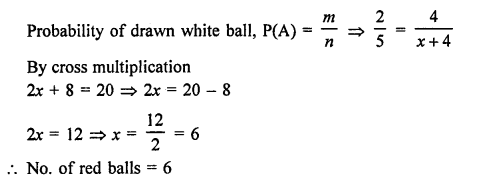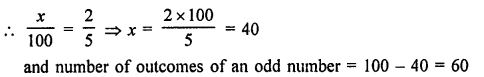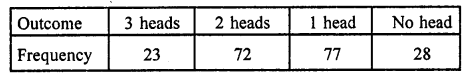# RD Sharma Class 9 Solutions Chapter 25 Probability VSAQS

## RD Sharma Class 9 Solutions Chapter 25 Probability VSAQS

These Solutions are part of RD Sharma Class 9 Solutions. Here we have given RD Sharma Class 9 Solutions Chapter 25 Probability VSAQS

Other Exercises

Question 1.
Define a trial.
Solution:
When we perform an experiment, it is called a trial of the experiment.

Question 2.
Define an elementary event.
Solution:
An outcome of a trial of an experiment is called an elementary event.

Question 3.
Define an event.
Solution:
An event association to a random experiment is said to occur in a trial.

Question 4.
Define probability of an event.
Solution:
In n trials of a random experiment if an event A happens m times, then probability of happening
of A is given by P(A) = $$\frac { m }{ n }$$

Question 5.
A bag contains 4 white balls and some red balls. If the probability of drawing a white ball from the bag is $$\frac { 2 }{ 5 }$$, find the number of red balls in the bag
Solution:
No. of white balls = 4
Let number of red balls = x
Then total number of balls (n) = 4 white + x red = (4 + x) ballsQuestion 6.
A die is thrown 100 times. If the probability of getting an even number is $$\frac { 2 }{ 5 }$$. How many times an odd number is obtained?
Solution:
Total number of a die is thrown = 100
Let an even number comes x times, then probability of an even number = $$\frac { x }{ 100 }$$Question 7.
Three coins are tossed simultaneously 200 times with the following frequencies of different outcomesFind the probability of getting at most two heads.
Solution:
Total number of three coins are tossed (n) = 200
Getting at the most 2 heads (m) = 72 + 77 + 28 = 177
Probability P(A) = $$\frac { m }{ n }$$ = $$\frac { 177 }{ 200 }$$

Question 8.
In the Q. No. 7, what is the probability of getting at least two heads?
Solution:
Total number of possible events = 200
No. of events getting at the least = 2 heads (m) = 23 + 72 = 95
Probability P(A) = $$\frac { m }{ n }$$ = $$\frac { 95 }{ 200 }$$ = $$\frac { 19 }{ 40 }$$

Hope given RD Sharma Class 9 Solutions Chapter 25 Probability VSAQS are helpful to complete your math homework.

If you have any doubts, please comment below. Learn Insta try to provide online math tutoring for you.